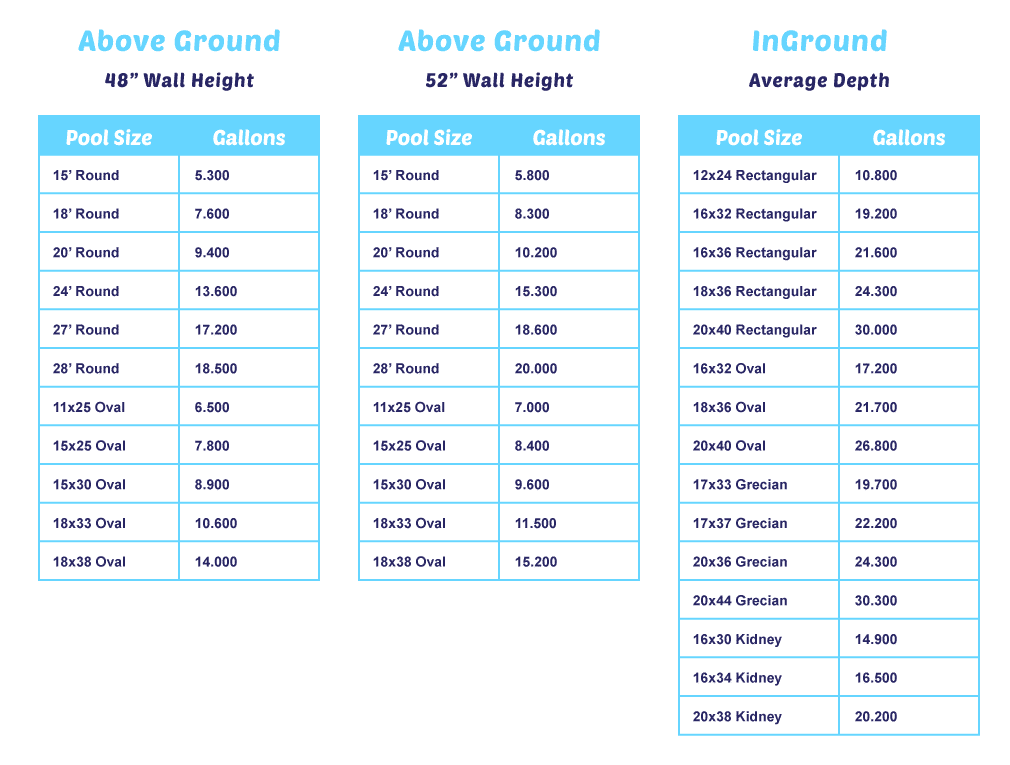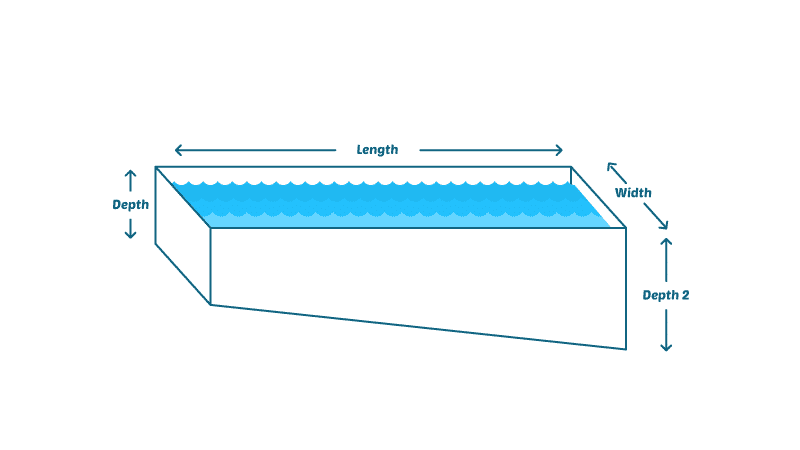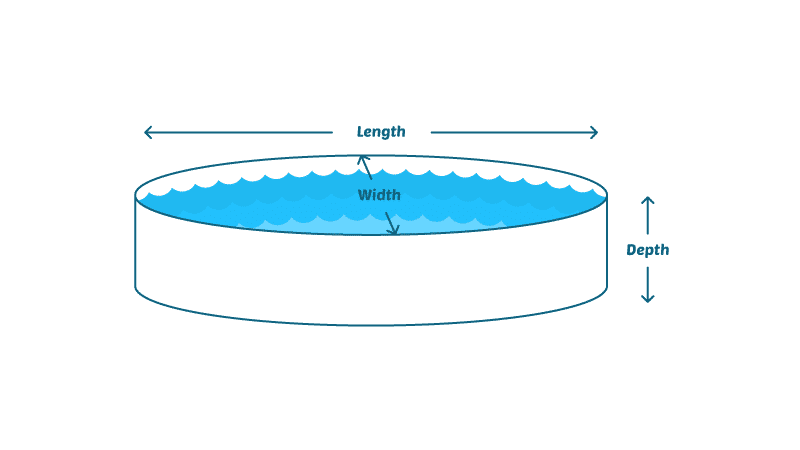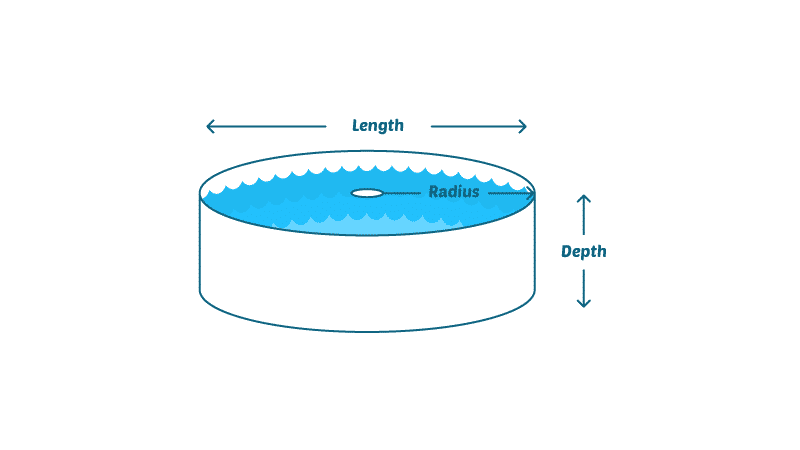# Pool Calculator: How Much Water Is In Your Pool?

Whoa, hold on there! Before you just go pouring chlorine and a bunch of other chemicals into your pool willy nilly, you need to know how much to use. It makes sense, right? When you do a small load of laundry, you only need a small amount of detergent. More clothes and more water require more detergent. It’s the same with your pool. The more water it holds, the more chemicals you’ll need to keep it clean and swimmable.

It’s always a good idea to follow the manufacturer’s instructions when adding chemicals to your pool water. But those instructions only work if you know how much water your pool holds. And if you don’t already know that, it’s time to break out the pool calculator.

(Oh, and let’s be clear, we don’t mean a pool chemical calculator. That’s an entirely different, but just as helpful tool.)

## A Pool Calculator Makes Your Life Easier

Luckily for you, lots of people have been maintaining pools for decades now. This means they’ve navigated the pitfalls and figured some things out so you don’t have to. That includes adding chemicals to your pool.

The necessary amounts of chlorine, pH increaser, shock, and any other chemical you use to care for your pool have all been figured out based on water volume. Even if it’s not just a rectangle or a circle, all you have to do is plug your pool’s measurements into a mathematical formula to get the volume.

But if you’re not into math or, as we like to call it, numerical torture, you can just as easily plug those numbers into a pool calculator and let it do the work for you.

Calcium Hypochlorite Shock
\$67.99
Use entire contents of bag when opened. If any granules settle to the bottom of the pool use brush to disperse. Add the recommended dosage of this product during evening hours while the filter pump is running.
If you click this link and make a purchase, we earn a commission at no additional cost to you. 08/12/2020 01:48 am

## How to Calculate Pool Volume

Before you do any math or use a pool calculator, you can also just use this quick-reference chart. Just be aware that the measurements and amounts may not be exact, depending on your pool’s size, and you’ll always get a better result if you measure your pool yourself.To use your pool’s exact measurements, you can also use our pool calculator. It rounds up just a bit, which will make chemical calculations easier. But don’t worry. It’s not enough to throw the chemistry balance off.

Pool Volume Calculator Choose the shape of your pool first.RectangleRound

Or, if you’re some kind of masochist math fan, you can do the calculations yourself based on the shape of your pool.

## Pool Volume Calculation Basics

In order to measure your pool’s volume, you’ll need a handful of basic equation elements.

A: Area
L: Length
W: Width
D: Depth
H: Height
V: Volume
d: Diameter
Pi: 3.14 constant

And just in case geometry class was a while ago for you, a few basic formulae to calculate area:

Area of a Square or Rectangle: A = L × W
Area of a Right Triangle: A = (L × W) ÷ 2
Area of a Circle: A = Pi × r2

In order to calculate cubic volume, you’ll need to include the pool’s depth as well as the surface area. To ensure those calculations are correct, divide your pool into sections by depth (shallow end, deep end).

Another key part of your equations is this: One cubic foot of water contains 7.5 gallons. So in order to determine the total number of gallons your pool holds, you’ll first determine the cubic feet measurement of your pool, then multiply that number by 7.5 to find the volume.

Now it’s just a matter of plugging in the numbers.

### Frustrated by adding chemicals and trying to keep your pool clear all the time?

We cut out all the confusion of pool maintenance in this easy-to-read illustrated ebook and video course. It'll help you save \$100 right away on pool care!

## Rectangular or Square Pool Volume

How you calculate volume will depend on how the bottom of your pool is laid out.

### Constant Depth

If your pool has only one depth, you’ll measure the length and width to calculate the surface area of the pool. Then you’ll multiply that number by the depth to calculate the volume in cubic feet. Then multiply that figure by 7.5 to calculate the volume of the pool in gallons.

L × W × D × 7.5 = V (in gallons)

So if your pool is 32 feet long, 16 feet wide, and 4 feet deep:

32 × 16 × 4 × 7.5 = 15,360 gallons

### Variable Depths with a Gradual Slope

If your pool has more than one depth (shallow end, deep end), you’ll need to do a little more math. First, determine the pool’s average depth. To do this, add the shallow end depth to the deep end depth, and divide by 2.(shallow D + deep D) ÷ 2 = average D (in feet)

For example, let’s say your pool’s shallow end is 3 feet, and the deep end is 9 feet:

(3 + 9) ÷ 2 = 6 feet (average depth)

Next, measure the length and width to calculate the surface area of the pool. Then you’ll multiply that number by the average depth to calculate the volume in cubic feet. Then multiply that figure by 7.5 to calculate the volume of the pool in gallons.

L × W × average D × 7.5 = V (in gallons)

Let’s assume your pool is 32 feet long, 16 feet wide, and has an average depth of 6 feet:

32 × 16 × 6 × 7.5 = 23,040 gallons

### Variable Depths with a Drop-Off

If, instead of a gradual slope down to the deep end, your pool has a definitive drop between the shallow and deep ends, the average depth will be different, even if the shallow end and deep end are still, for example, 3 feet and 9 feet, respectively.

It may be easier, in this case, to treat the shallow and deep ends as separate pools. This will allow you to apply the volume of each section using the constant depth formula.

Once you have the volumes of both parts, simply add them together to determine the volume for the whole pool.

Measuring Tape Reel - 200 ft. / 60 m.
\$25.64
If you click this link and make a purchase, we earn a commission at no additional cost to you. 08/12/2020 07:48 am

## Round Pool Volume

To find a round pool’s volume in gallons, you’ll multiply Pi by the radius squared, by the depth, by 7.5. It looks like this:

3.14 × r2 × D × 7.5 = V (in gallons)

But before you can solve that equation, you need to find the radius of the pool.

Start by measuring your pool’s diameter, which is measured at its widest point. You can also call this its width or length. On a round pool, they’re all the same number.Then divide the diameter by 2 to get the radius:

d ÷ 2 = r

Let’s say your pool measures 24 feet in diameter.

24 ÷ 2 = 12r × r = r2

12 × 12 = 144

Next, measure the pool’s depth. Let’s say it’s 4 feet deep.

So to find the volume, we go back to the original equation:

3.14 × r2 × D × 7.5 = V (in gallons)

So the round pool’s volume is:

3.14 × 144 × 4 × 7.5 = 13,564.8 gallons

Whew! Anyone else have a headache? This is actually an easy equation with (mostly) whole numbers and exact measurements. But what if your pool’s measurements aren’t exact?

## Uneven Measurements

It’s possible that your pool’s dimensions won’t be round numbers. There may be an extra inch here and there. To make calculations easier on yourself, round each measurement off to the nearest foot.

One inch is equal to 0.0833 feet. Multiply that number by the number of inches in your measurement to arrive at a percentage of one foot.

So if your pool measures 31 feet, 9 inches in length:

9 inches × 0.0833 = 0.7497 feet

Round that up and add it to your pool’s length measurement in feet.

31 + 0.75 = 31.75 feet

Then you can use that number in the volume-finding equations.

## Irregularly Shaped Pool Volume

Not all pools are rectangular, square, or round. Some are oval, others are the classic kidney shape, and then some just wiggle all over the place, mimicking natural shorelines.

If you have an irregularly shaped pool, you can still calculate the volume. You’ll just have to do—you guessed it—even more math!

The easiest way to calculate an oddly shaped pool is to imagine it broken down into two or more smaller, regularly shaped parts. Try to see smaller, individual squares, rectangles, or circles within the larger, irregular shape.

Once you’ve mapped them out, measure them, and then use the same formulae that apply to those regular shapes to calculate the volume in each smaller section. Then just add all the volume figures together, and you’ll have a pretty close approximation of the pool’s total volume.

Admittedly, this could also be known as the “winging it” procedure, and you’re not going to get a completely accurate volume. If your pool is shaped like a fractal, your best bet is to have a professional take the measurements for you.

## Done With Numbers? Call in a Pro!

Are you at the point where if you have to do one more math problem, you’re going to just cover the pool and call it a year? We hear you.

If a pool volume chart or pool calculator doesn’t work properly for you because you have an unusual pool shape, or you just want everything to be accurate to the decimal point, you can have a pool professional take the measurements and figure out the volume for you.

## How Does Your Pool Measure Up?

Have you realized that you’ve been adding too much chlorine to your pool? Not enough shock? So much salt that you can no longer submerge? Yup, using a pool calculator will do that for you.